## Dividing Radical Expressions

When dividing radical expressions, use the quotient rule.

For all real values, a and b, b ≠ 0

1. If n is even, and a ≥ 0, b > 0, then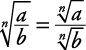2. If n is odd, and b ≠ 0, thenThat's a mathematical symbols way of saying that when the index is even there can be no negative number in the radicand, but when the index is odd, there can be.

Radical expressions are written in simplest terms when

• The index is as small as possible.

• The radicand contains no factor (other than 1) which is the nth or greater power of an integer or polynomial.

• The radicand contains no fractions.

• No radicals appear in the denominator.

##### Example 1

Simplify each of the following.

1.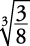2.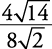1. Using the quotient rule for radicals,2. Using the quotient rule for radicals,## Rationalizing the denominator

An expression with a radical in its denominator should be simplified into one without a radical in its denominator. This process is called rationalizing the denominator. This is accomplished by multiplying the expression by a fraction having the value 1, in an appropriate form.

##### Example 2

Simplify each of the following.

1.2.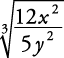1. To rationalize this denominator, the appropriate fraction with the value 1 is, since that will eliminate the radical in the denominator, when used as follows:Note we elected to find's principal root.

2.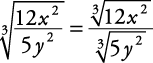What can be multiplied with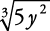so the result will not involve a radical? The answer is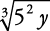or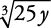. That choice is made so that after they are multiplied, everything under the radical sign will be perfect cubes.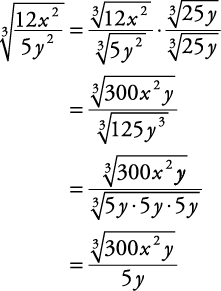## Conjugates

If a and b are unlike terms, then the conjugate of a + b is ab, and the conjugate of ab is a + b. The conjugate of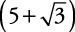is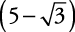. Conjugates are used for rationalizing the denominator when the denominator is a two‐termed expression involving a square root.

##### Example 3

Simplify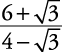.

To rationalize the denominator of this expression, multiply by a fraction in the form of the denominator's conjugate over itself.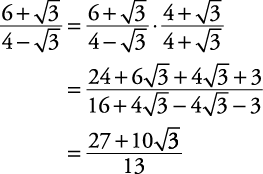Back to Top
A18ACD436D5A3997E3DA2573E3FD792A